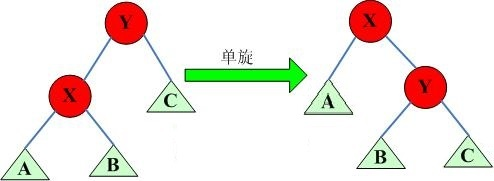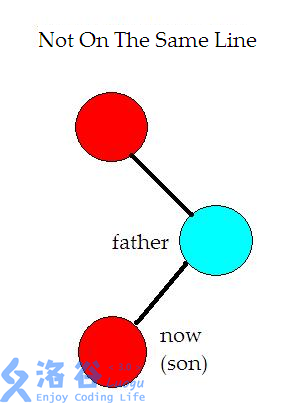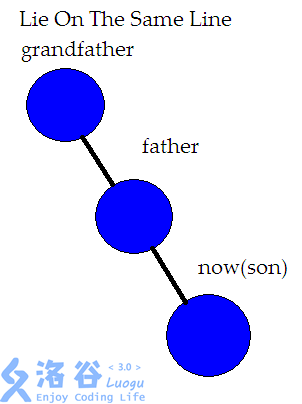# 引言

## 一.旋转是个什么东西？？？$Y>X,B>X,$ 如果 $Y<B,$ 那么显然B不会跑到左子树去，所以得出结论: $$Y>B$$

### 我们定义一个结点与他父亲的关系是 $x$ ,那么在旋转时,他的父亲成为了他的 $!x$ 儿子,并且那个上文中所说的“多余结点”，同时也是当前节点的 $!x$ 儿子，但在旋转之后需要成为当前节点的“前”父节点的 $x$ 儿子。

$Talk$ $is$ $\color{silver}{cheap}$ $,show$ $you$ $the$ $\color{silver}{code}$ :

inline void update(int x){
if(x){
sub_size[x]=cnt[x];
if(sons[x])sub_size[x]+=sub_size[sons[x]];
if(sons[x])sub_size[x]+=sub_size[sons[x]];
}
return ;
}
inline bool get_which(int x){
return sons[f[x]]==x;
}
inline void rotate(int x){
int father=f[x],g_father=f[father];
bool which_son=get_which(x);//当前节点的关系
sons[father][which_son]=sons[x][which_son^1];
f[sons[father][which_son]]=father;
sons[x][which_son^1]=father;
f[father]=x;
f[x]=g_father;
if(g_father){
sons[g_father][get_which(father)]=x;
}
update(x);
update(father);
}

$son$ 表示每个节点的左右儿子， $f$ 表示每个节点的父亲 $sub\_size[i]$ 表示以 $i$ 为根的子树的大小。

$qwqqq$ 是为了方便执行之后的 $zz$ 操作啊

$\mathcal{1.}$ 如果爷爷节点、父节点与自己不共线，那么就是这样$\mathcal{2.}$ 如果三个节点共线的话，那么就先要旋转父节点，因为如果先旋转子节点的话，我们就会发现原来华丽的一条链的结构被破坏，接下来的一系列操作即会导致这棵树失衡，所以应该先旋转父节点，再旋转子节点 $qwq$╮(￣▽￣")╭虽然我不是很想做效果图，但是为了你们我忍了（逃

$emmmm$ 实质上就是说，我们在链很长的时候，每次执行先旋父节点再旋当前节点的操作，一次总操作之后，这条链的深度会减半。

$Talk$ $is$ $\color{silver}{cheap}$ $,show$ $you$ $the$ $\color{silver}{code}$ :

inline void splay(int x){
for (int fa;fa=f[x];rotate(x))
if (f[fa])
rotate((get_which(x)==get_which(fa))?fa:x);
root=x;
}

1.每次进行有关点的操作时都要 $Splay$ 一次，因为要维护树的随机性

2.注意第一条中的“有关点”，比如当给出排名找数的时候，由于其实跟这个点没什么关系，所以不用 $Splay$ .

$Show$ $The$ $Whole$ $Code$ ：

// luogu-judger-enable-o2
#include<iostream>
#include<cstdio>
using namespace std;
#define MAXN 1000000
int f[MAXN],cnt[MAXN],value[MAXN],sons[MAXN],sub_size[MAXN],whole_size,root;
int res=0,k=1;
char c=getchar();
while(!isdigit(c)){
if(c=='-')k=-1;
c=getchar();
}
while(isdigit(c)){
res=(res<<1)+(res<<3)+c-48;
c=getchar();
}
return res*k;
}
inline void S_Clear(int x){
sons[x]=sons[x]=f[x]=sub_size[x]=cnt[x]=value[x]=0;
}
inline bool get_which(int x){
return sons[f[x]]==x;
}
inline void update(int x){
if (x){
sub_size[x]=cnt[x];
if (sons[x]) sub_size[x]+=sub_size[sons[x]];
if (sons[x]) sub_size[x]+=sub_size[sons[x]];
}
return ;
}
inline void rotate(int x){
int father=f[x],g_father=f[father],which_son=get_which(x);
sons[father][which_son]=sons[x][which_son^1];
f[sons[father][which_son]]=father;
sons[x][which_son^1]=father;
f[father]=x;
f[x]=g_father;
if(g_father){
sons[g_father][sons[g_father]==father]=x;
}
update(father);
update(x);
}
inline void splay(int x){
for (int fa;fa=f[x];rotate(x))
if (f[fa])
rotate((get_which(x)==get_which(fa))?fa:x);
root=x;
}
inline void insert(int x){
if(!root){
whole_size++;
sons[whole_size]=sons[whole_size]=f[whole_size]=0;
root=whole_size;
sub_size[whole_size]=cnt[whole_size]++;
value[whole_size]=x;
return ;
}
int now=root,fa=0;
while(1){
if(x==value[now]){
cnt[now]++;
update(now);
update(fa);
splay(now);
break;
}
fa=now;
now=sons[now][value[now]<x];
if(!now){
whole_size++;
sons[whole_size]=sons[whole_size]=0;
f[whole_size]=fa;
sub_size[whole_size]=cnt[whole_size]=1;
sons[fa][value[fa]<x]=whole_size;
value[whole_size]=x;
update(fa);
splay(whole_size);
break;
}
}

}
inline int find_num(int x){
int now=root;
while(1){
if(sons[now]&&x<=sub_size[sons[now]])
now=sons[now];
else {
int temp=(sons[now]?sub_size[sons[now]]:0)+cnt[now];
if(x<=temp)return value[now];
x-=temp;
now=sons[now];
}
}
}

inline int find_rank(int x){
int now=root,ans=0;
while(1){
if (x<value[now])
now=sons[now];
else{
ans+=(sons[now]?sub_size[sons[now]]:0);
if (x==value[now]){
splay(now); return ans+1;
}
ans+=cnt[now];
now=sons[now];
}
}
}
inline int find_pre(){
int now=sons[root];
while(sons[now])now=sons[now];
return now;
}
inline int find_suffix(){
int now=sons[root];
while(sons[now])now=sons[now];
return now;
}
inline void my_delete(int x){
int hhh=find_rank(x);
if (cnt[root]>1){
cnt[root]--;
update(root);
return;
}
if (!sons[root]&&!sons[root]) {
S_Clear(root);
root=0;
return;
}
if (!sons[root]){
int old_root=root;
root=sons[root];
f[root]=0;
S_Clear(old_root);
return;
}

else if (!sons[root]){
int old_root=root;
root=sons[root];
f[root]=0;
S_Clear(old_root);
return;
}
int left_max=find_pre(),old_root=root;
splay(left_max);
sons[root]=sons[old_root];
f[sons[old_root]]=root;
S_Clear(old_root);
update(root);
}

int main(){
int m,num,be_dealt;
cin>>m;
for(int i=1;i<=m;i++){
switch(num)
{
case 1:insert(be_dealt);break;
case 2:my_delete(be_dealt);break;
case 3:printf("%d\n",find_rank(be_dealt));break;
case 4:printf("%d\n",find_num(be_dealt));break;
case 5:insert(be_dealt);printf("%d\n",value[find_pre()]);my_delete(be_dealt);break;
case 6:insert(be_dealt);printf("%d\n",value[find_suffix()]);my_delete(be_dealt);break;
}
}
return 0;
}

2018-04-22 20:56:29 in 数据结构学习